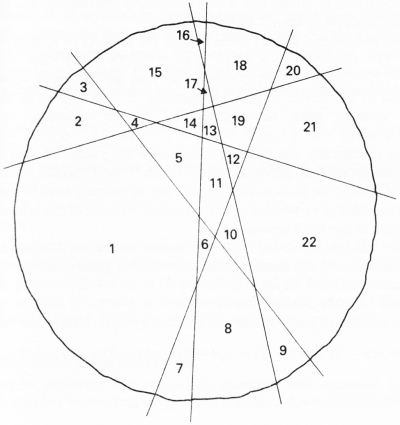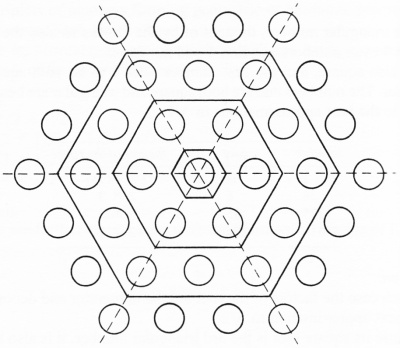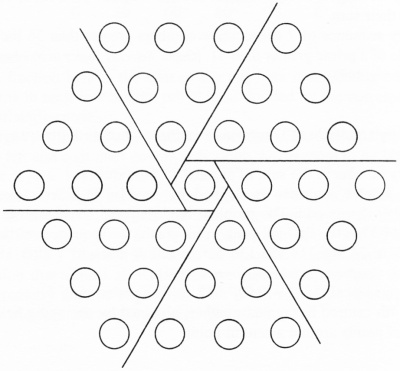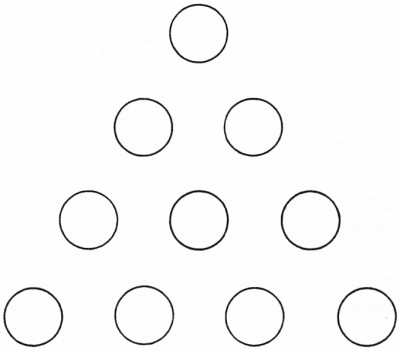## Polygonal Numbers and Pie

### November 21st, 2007

Four mathematical illustrations from Wells’ Dictionary of Curious and Interesting Numbers.

“The maximum number of pieces into which a pancake [or pie] can be cut with 6 slices” is 22 (p78):22 is also the 4th pentagonal number — whereas “the 4th centered hexagonal number, obtained by arranging hexagonal layers around a central point,” is 37 (p99):“By a different division [of the above] the nth centered hexagonal number is equal to 6Tn-1 + 1, where Tn is the nth triangular number” (p100). Here we see a composition using the 3rd triangular number:And the 4th triangular number? — 10, the tetractys, a figuration “so holy [to the Pythagoreans] that they even swore oaths by it” (p61, c.f. the obverse seal):### 2 Responses to “Polygonal Numbers and Pie”

1. Mirco Says:

Nice diagrams.

Prophecy: this century will rediscover the central role of figurate numbers, particularly in the physical sciences (Pythagoras vindicated!).

Till then, we can already use them as Nature’s built-in Yantras

2. Mirco2 Says:

Nice diagrams.

Prophecy: this century will rediscover the central role of figurate numbers, particularly in the physical sciences (Pythagoras vindicated!).

Till then, we can already use them as Nature’s built-in Yantras